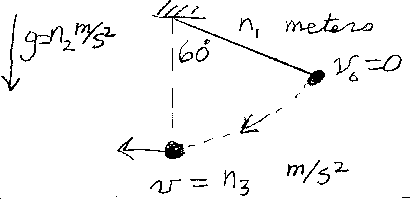Problem C4: A simple pendulum of length n1 meters is let go from rest at an angle of 60 degrees. The pendulum is on a strange planet with an acceleration due to gravity of g=n2 meters/sec2. What is the speed of the bob at the bottom of the pendulum where it is a maximum. If the speed at the bottom is v=n3 meters/sec, What is n3?n1 = n2 = Input n3:
If you are currently in my class, you can record your grade by entering your name and student ID number (without the leading zeros) below and clicking on "record grade".
 First Name = Last Name = ID = Problem: